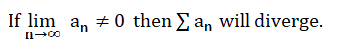# Divergent Series

A divergent series doesn’t have a limit. In other words, it never settles on a certain number. Instead, it usually goes towards infinity. For example, the series

1 + 2 + 3…

will keep on growing to infinity.

You don’t have to sum the whole series to show it’s divergent: you use a technique called partial sums, where you add up some of the terms (e.g. the first n terms).

## Problems with Divergent Series

All divergent series usually add up to infinity. On the other hand, as they add up to infinity, so you could argue that they do converge (if you consider infinity to be a number). This mathematical conundrum may have been one reason why they were disliked to the extreme in the early days of their formulation (although back in the day, they lacked some clear definitions of what divergence actually meant).

Nineteenth century Norwegian mathematician Niels Henrik Abel called divergent series “the invention of the devil” (cited in Young, 1992), in part because strange things happen when you take partial sums.

For example, I stated above that the sum of all natural numbers (whole, non-negative numbers that we use to count) is infinity. However, you can show, with partial sums, that 1 + 2 + 3 + … = −1/12.

That bizarre result is actually used in various contexts, including string theory. The proof is beyond the scope of this article, but if you’re interested, watch this numberphile video:

## Series that Diverge

Some series are well known to diverge. For example, 1 + 1 + 1… is a simple series that diverges.

The power series diverges for large values of n (although it converges for intervals of x (MIT, 2020).

Other series oscillate, like 1 – 1 + 1 – 1…. These oscillating series are also considered divergent (or in some cases, partially divergent). The partial sums do have an average value of ½, but that doesn’t equal convergence.

## Test for Divergent Series

You don’t usually show that a series is divergent. Instead, you show it isn’t convergent. There is an exception: the divergence test, which states that a series is guaranteed to diverge if the terms in the series don’t go towards zero in the limit:The test is rather awkward to use, because it says nothing about divergence or convergence if the terms do go to zero. If this happens, the series may converge, or it may diverge, there’s no way to tell without performing a different series convergence test.

## References

MIT (2020). Online Calculus Textbook.
Stewart, J. (2007). Calculus. Thomas Brooks/Cole; 6th edition.
Young, R. (1992). Excursions in Calculus: An Interplay of the Continuous and the Discrete, Volume 13. Cambridge University.

CITE THIS AS:
Stephanie Glen. "Divergent Series" From StatisticsHowTo.com: Elementary Statistics for the rest of us! https://www.statisticshowto.com/divergent-series/